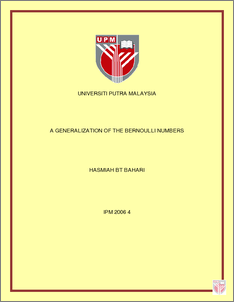# A Generalization of the Bernoulli Numbers

## Citation

Bahari, Hasmiah (2006) A Generalization of the Bernoulli Numbers. Masters thesis, Universiti Putra Malaysia.

## Abstract

The Bernoulli numbers are among the most interesting and important number sequences in mathematics. It plays an important and quite mysterious role in various places like number theory, analysis and etc. In general, many existing generalizations of Bernoulli numbers { for example []20re based on consideration of more general forms for the left side of the following equality }nB,21 ()Σ∞==−0!1expnnnntBtt or for some related functions. In this study, a generalization of Bernoulli numbers is offered by the use of their relations with Pascal’s triangle. The thesis begins with the generalization of Bernoulli numbers {} then a representation of is presented, followed by the proof of the main result for odd n case (even case of n was considered in ∞=1nnBnB[]2 ). Then special cases of Bernoulli numbers, namely when the initial sequence is an geometric or arithmetic sequence, are considered. In these special cases more detailed representations of are obtained. Then irreducibility problem over Z of polynomials closely related to is considered followed by solution of this problem for some values of . At the end some unsolved problems, with which we have come across in doing this thesis, over the field nBnBnZ are formulated.Preview
PDF
IPM_2006_4.pdfView Item